# How to Calculate and Solve for Gas Recovery Factor (Production) | Reservoir Fluid Flow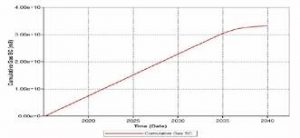The image above represents a gas recovery factor (G).

To compute for a gas recovery factor (production), two essential parameters are needed and these parameters are cumulative gas production (GP) and initial gas in place (G).

The formula for calculating the gas recovery factor (G):

RFG = Gp / G

Where:

RFG = Gas Recovery Factor (G)
GP = Cumulative Gas Production
G = Initial Gas in Place

Let’s solve an example;
Find the gas recovery factor (G) when the cumulative gas production is 13 and the initial gas in place is 17.

This implies that;

GP = Cumulative Gas Production = 13
G = Initial Gas in Place = 17

RFG = Gp / G
RFG = 13 / 17
RFG = 0.764

Therefore, the gas recovery factor (G) is 0.764.

Calculating the Cumulative Gas Production when the Gas Recovery Factor (G) and the Initial Gas in Place is Given.

GP = RFG x G

Where;

GP = Cumulative Gas Production
RFG = Gas Recovery Factor (G)
G = Initial Gas in Place

Let’s solve an example;
Find the cumulative gas production when the gas recovery factor (G) is 24 and the initial gas in place is 6.

This implies that;

RFG = Gas Recovery Factor (G) = 24
G = Initial Gas in Place = 6

GP = RFG x G
GP = 24 x 6
GP = 144

Therefore, the cumulative gas production is 144.

Calculating the Initial Gas in Place when the Gas Recovery Factor (G) and the Cumulative Gas Production is Given.

G = GP / RFG

Where;

G = Initial Gas in Place
RFG = Gas Recovery Factor (G)
GP = Cumulative Gas Production

Let’s solve an example;
Find the initial gas in place when the gas recovery factor (G) is 30 and the cumulative gas production is 60.

This implies that;

RFG = Gas Recovery Factor (G) = 30
GP = Cumulative Gas Production = 60

G = GP / RFG
G = 60 / 30
G = 2

Therefore, the initial gas in place is 2.

Nickzom Calculator – The Calculator Encyclopedia is capable of calculating the gas recovery factor (G).

To get the answer and workings of the gas recovery factor (G) using the Nickzom Calculator – The Calculator Encyclopedia. First, you need to obtain the app.

You can get this app via any of these means:

To get access to the professional version via web, you need to register and subscribe for NGN 1,500 per annum to have utter access to all functionalities.
You can also try the demo version via https://www.nickzom.org/calculator

Apple (Paid) – https://itunes.apple.com/us/app/nickzom-calculator/id1331162702?mt=8
Once, you have obtained the calculator encyclopedia app, proceed to the Calculator Map, then click on Petroleum under EngineeringNow, Click on Reservoir Fluid Flow under Petroleum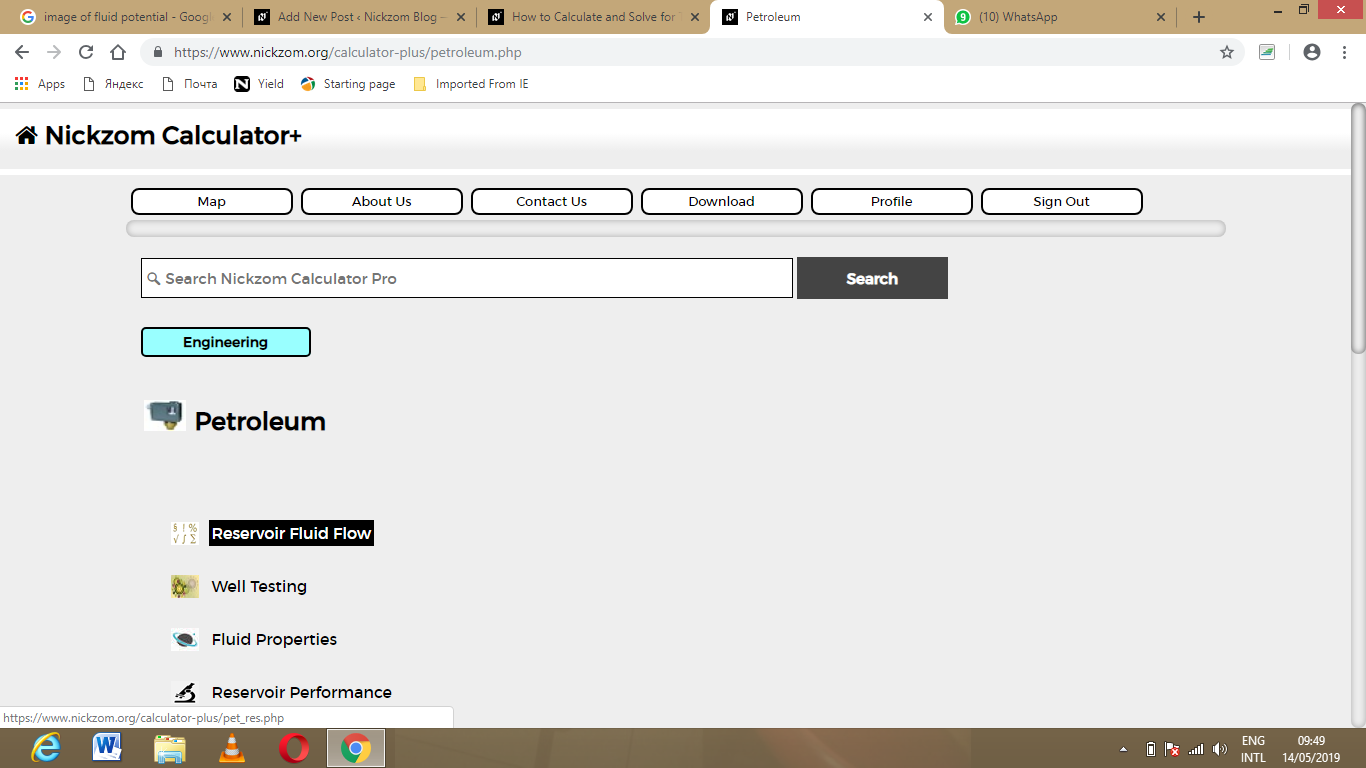Now, Click on gas recovery factor (G) under Reservoir Fluid Flow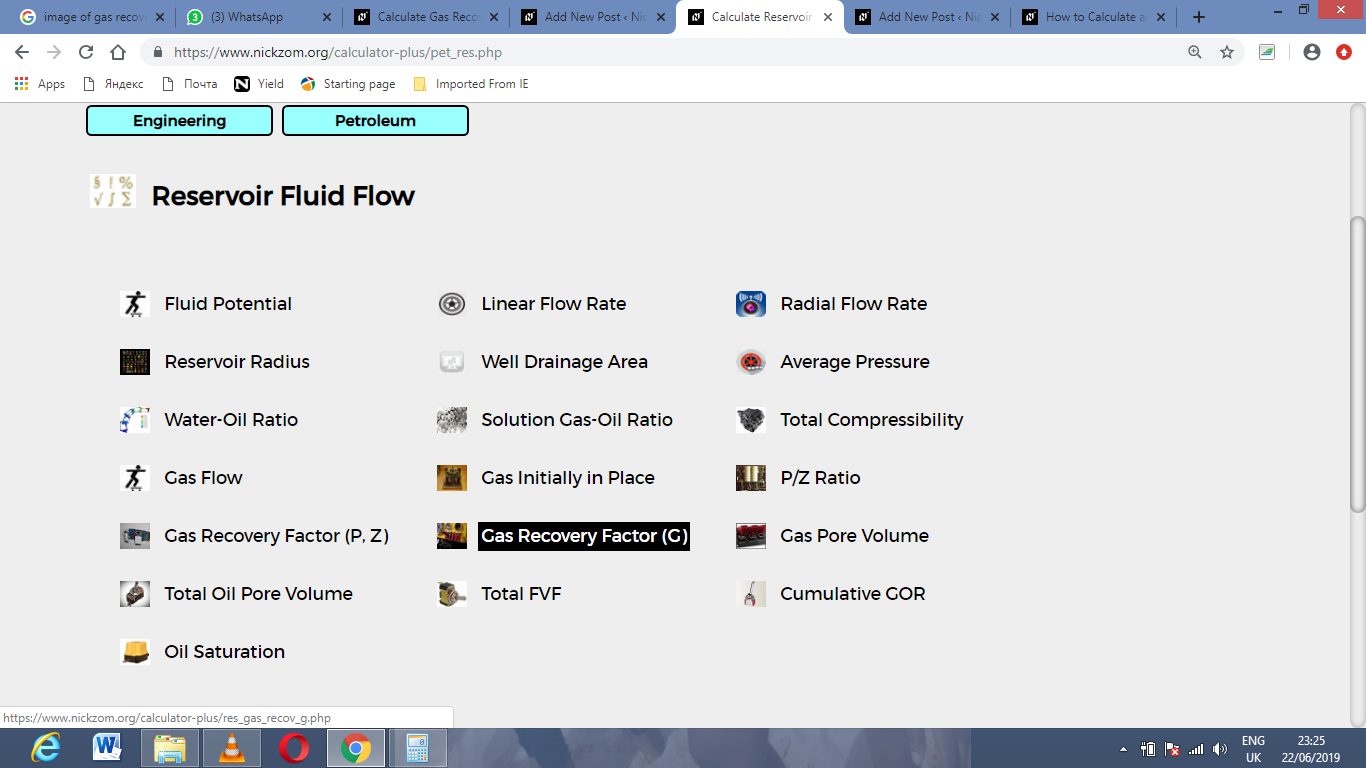The screenshot below displays the page or activity to enter your value, to get the answer for the gas recovery factor (G) according to the respective parameter which are the cumulative gas production (GP) and initial gas in place (G).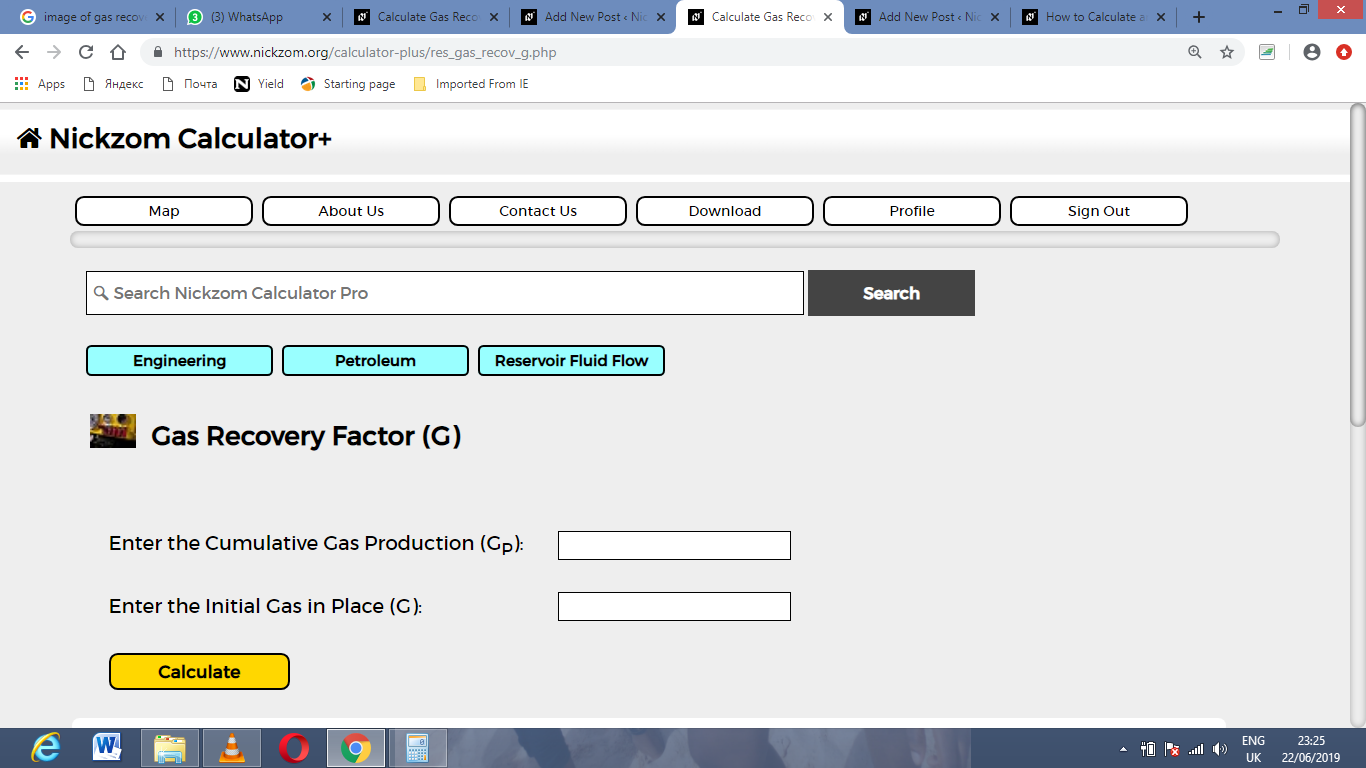Now, enter the value appropriately and accordingly for the parameter as required by the cumulative gas production (GP) is 13 and initial gas in place (G) is 17.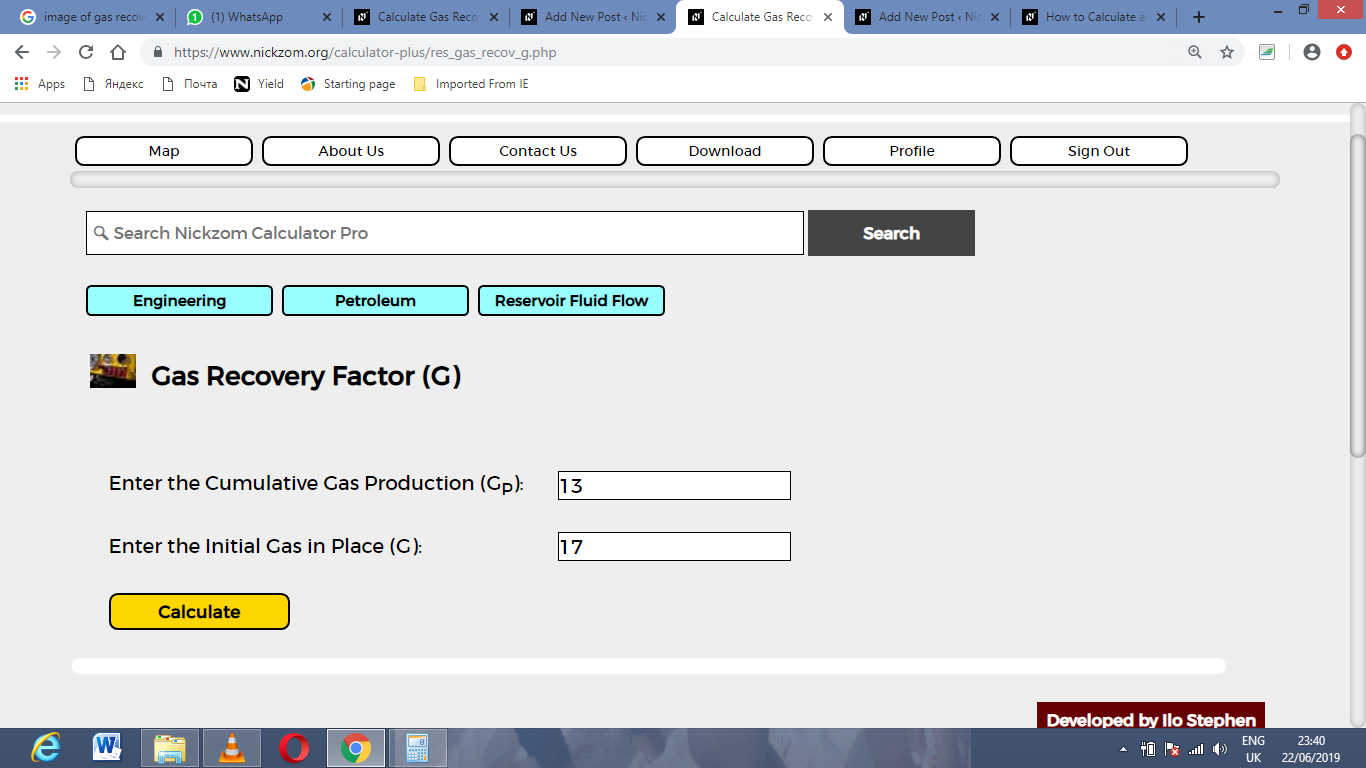Finally, Click on Calculate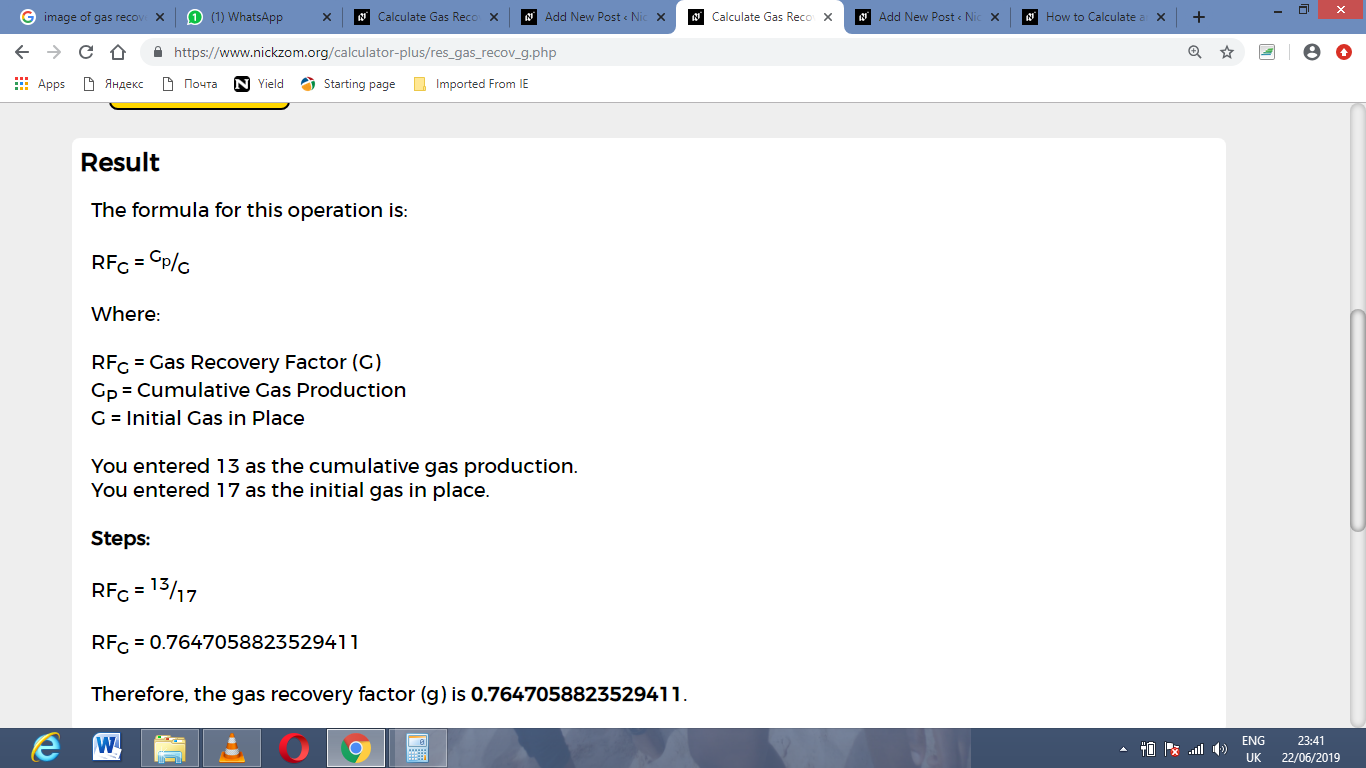As you can see from the screenshot above, Nickzom Calculator– The Calculator Encyclopedia solves for the gas recovery factor (G) and presents the formula, workings and steps too.